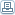# Some Basic Concepts of Fiber Optic Loss Testing

 By: Colin YaoWhen testing loss in a fiber optic link, some basic principles must be kept in mind all the time.1. The testing wavelength should always be the same as the working wavelength. Because optical fiber loss varies with light wavelength, you will get incorrect result if your measuring wavelength is different from the actual working wavelength. For example, if a system is designed for 1550nm but you test it with 1310nm light source and power meter, the result will not be correct.2. The testing light source should be the same as the intended working lightwave equipment light source. If the system is designed for a LED source, you should test it with a LED source. If the system is designed for multimode laser light, you should use a multimode laser light source for testing. This is also true for single mode laser light source.Fiber optic equipment used in a loss testingIn a basic loss testing setup, four types of test equipment are needed. They are the light source, the power meter, the reference patch cables and the adapter (mating sleeve).Here are some considerations when choosing your equipment.The light source should have the same wavelength as the operating equipment, proper mode (multimode or single mode, should be same as the operating equipment), type (LED or laser, same as the operating equipment) and proper connector.The power meter should have the same wavelength as the light source, proper connector and calibrated.The reference patch cables should be high quality with know loss, proper connectors and be the same type as the fiber plant being tested.The adapter (mating sleeve) should be with high quality ceramic sleeves and be proper type (FC, SC, LC, etc).Understanding dB (decibel) in fiber optic loss testingAs in any power measurement, fiber optic light power measurement unit can be expressed in milliwatt (mW), but a more convenient unit is dB(decibel).Decibel (dB) is most often used in electronics testing. It is the ratio between two levels. One level is the input and the other level is the output. The ratio is calculated in logarithmic as explained below.For power measurement, dB is defined as: dB = 10 x log(output power/input power)So for example, after a fiber link, the output light power level becomes 50% of its input, the loss of the link will be 10log(0.5)= -3 dB.Since dB is actually a ratio, it has no absolute units. So from above measurement sample, we have no idea of the actual power, may it be 0.1 mW or 1 mW.That is why we have another unit dBm. It is the ratio of the measured power to 1mW of reference power. It is defined as: dBm = 10xlog(measured power/1mW)So for example, a 0.1mW light power expressed in dBm will be 10xlog(0.1mW/1mW)=-10 dBm.From above we know that dBm is a absolute unit, we know exactly how many mW it is.For fiber optic loss testing, decibel is the most often used unit since it is much easier to work with. Why? Because two dB values can be simply added or subtracted. For example, a total fiber link may have three sections, each has loss of 0.5dB, 5dB and 0.5dB. The total loss can then be easily concluded as 0.5dB + 5dB + 0.5dB = 6 dB. You can try to convert it to actual milliwatt and you will see that I am right!
 Computers